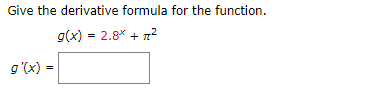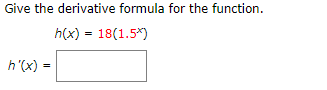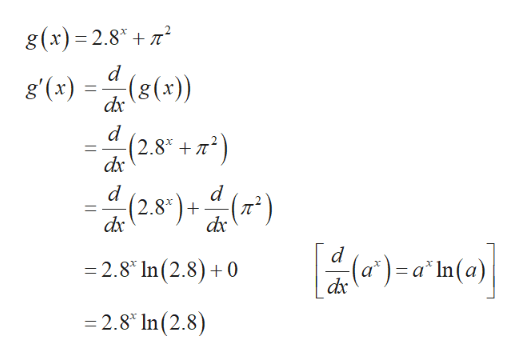# Give the derivative formula for the functiong(x) = 2.8*g '(x) Give the derivative formula for the function.h(x) 18(1.5*)h'(x)

Question
1 viewshelp_outlineImage TranscriptioncloseGive the derivative formula for the function g(x) = 2.8* g '(x) fullscreenhelp_outlineImage TranscriptioncloseGive the derivative formula for the function. h(x) 18(1.5*) h'(x) fullscreen
check_circle

Step 1

Problem 1:

To find the determine the deri...help_outlineImage Transcriptioncloseg(x)= 2.8 2 d g'(x) (s(x)) (2.8* d (2.8) (#*) dx dx d =2.8 In(2.8) + 0 dx =2.8 In (2.8) fullscreen

### Want to see the full answer?

See Solution

#### Want to see this answer and more?

Solutions are written by subject experts who are available 24/7. Questions are typically answered within 1 hour.*

See Solution
*Response times may vary by subject and question.
Tagged in

### Derivative